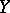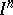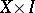Absolute retract for normal spaces

(diff) ← Older revision | Latest revision (diff) | Newer revision → (diff)
A topological spacesuch that every mappingof any closed subsetof an arbitrary normal spacecan be extended to the entire space. A direct product of absolute retracts for normal spaces is an absolute retract for normal spaces, as is any retract (cf. Retract of a topological space) of an absolute retract for normal spaces. In particular, the following spaces are absolute retracts for normal spaces: the unit interval; the-dimensional cube; and the Hilbert cube. Any two mappings of a binormal space into an absolute retract for normal spaces are homotopic; while a binormal absolute retract for normal spaces is contractible into a point.
A binormal space is a spacefor which the productis normal. It can be proved that a spaceis binormal if and only ifis normal and countably paracompact (cf. Paracompactness criteria).